##### Statistics For Dummies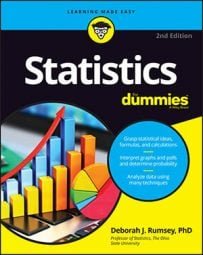After you identify that a random variable X has a binomial distribution, you'll likely want to find probabilities for X. The good news is that you don't have to find them from scratch; you get to use established statistical formulas for finding binomial probabilities, using the values of n and p unique to each problem.

Probabilities for a binomial random variable X can be found using the following formula for p(x):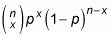where

• n is the fixed number of trials.

• x is the specified number of successes.

• nx is the number of failures.

• p is the probability of success on any given trial.

• 1 – p is the probability of failure on any given trial. (Note: Some textbooks use the letter q to denote the probability of failure rather than 1 – p.)

These probabilities hold for any value of X between 0 (lowest number of possible successes in n trials) and n (highest number of possible successes).

The number of ways to rearrange x successes among n trials is called "n choose x," and the notation is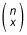It's important to note that this math expression is not a fraction; it's math shorthand to represent the number of ways to do these types of rearrangements.

In general, to calculate "n choose x," you use the following formula: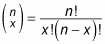The notation n! stands for n-factorial, the number of ways to rearrange n items. To calculate n!, you multiply n(n – 1)(n – 2) . . . (2)(1). For example, 5! is 5(4)(3)(2)(1) = 120; 2! is 2(1) = 2; and 1! is 1. By convention, 0! equals 1.

Suppose you have to cross three traffic lights on your way to work. Let X be the number of red lights you hit out of the three. How many ways can you hit two red lights on your way to work? (For this example, you can assume that a yellow light equates to a red light.) Well, you could hit a green one first, then the other two red; or you could hit the green one in the middle and have red ones for the first and third lights, or you could hit red first, then another red, then green. Letting G = green and R=red, you can write these three possibilities as: GRR, RGR, RRG. So you can hit two red lights on your way to work in three ways, right?

Check the math. In this example, a "trial" is a traffic light; and a "success" is a red light. (Yes, that seems weird, but a success is whatever you are interested in counting, good or bad.) So you have n = 3 total traffic lights, and you're interested in the situation where you get x = 2 red ones. Using the fancy notation,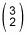means "3 choose 2" and stands for the number of ways to rearrange 2 successes in 3 trials.

To calculate "3 choose 2," you do the following: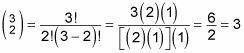This confirms the three possibilities listed for getting two red lights.

Now suppose the lights operate independently of each other and each one has a 30% chance of being red. Suppose you want to find the probability distribution for X. (That is, a list of all possible values of X — 0,1,2,3 — and their probabilities.)

Before you dive into the calculations, you first check to see if you have a binomial situation here. You have n = 3 trials (traffic lights) — check. Each trial is success (red light) or failure (yellow or green light; in other words, "non-red" light) — check. The lights operate independently, so you have the independent trials taken care of, and because each light is red 30% of the time, you know p = 0.30 for each light. So X = number of red traffic lights has a binomial distribution. To fill in the nitty gritties for the formulas, 1 – p = probability of a non-red light = 1 – 0.30 = 0.70; and the number of non-red lights is 3 – X.

Using the formula for p(x), you obtain the probabilities for x = 0, 1, 2, and 3 red lights: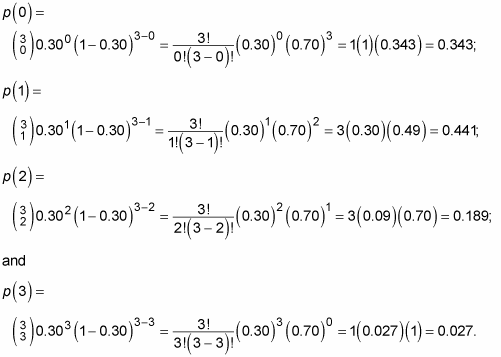The final probability distribution for X is shown in the following table. Notice these probabilities all sum to 1 because every possible value of X is listed and accounted for.

Probability Distribution for X = Number of Red Traffic Lights (n = 3, p = 0.30):

X p(x)
0 0.343
1 0.441
2 0.189
3 0.027